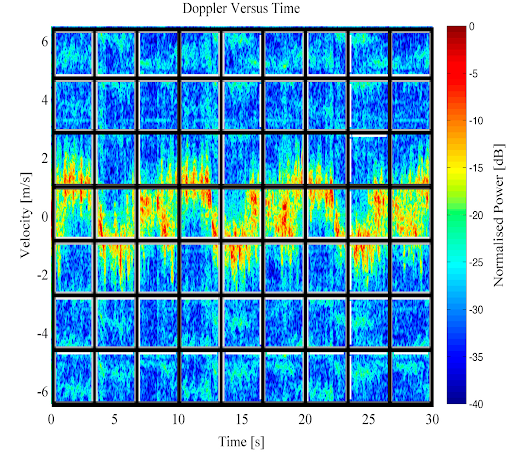•written on Dec 23, 2019
by Martin Rupp

Artificial Intelligence: A Short Overview of Classification Techniques for RADAR Targets through Neural Networks (1/2)

ATC-Training Artificial IntelligenceFast reaction and decision-making of the RADAR operator is a key factor in ATC. There is a need to develop techniques for the automatic extraction and fine-granulated classification of RADAR objects, allowing for faster and better decision making and more effective processes.

In this article we aim at presenting an overview of the techniques used for such classification by the neural networks. We assume the reader to be familiar with basic terminology of the neural networks (see previous articles of this series).

Introduction to Multiple Layers Neural Networks

Efficient Neural networks are multiple layers of neural networks,  also called Deep Learning neural networks. In such systems, each layer of neuron have a specific role which will depend on the design and the goal which is targeted. A typical Multi-layer neural network can have dozens of layers.The number of layers is called the depth of a neural network.A layer can perform for example one of the following operations

• Filtering;
• Detecting (Patterns) ;
• Extracting (Patterns) ;
• Subsampling;
• Merging (fusion);
• Fuzzing;
• Pooling & Max-Pooling;
• Convolution;
• Classification (usually output layer);
• Regression (usually output layer).

Each layer has a given number of neurons, called the width of the neural network.

The total amount of neurons is called the size of the neural network.

Given a classification problem, it is needed to define the amount of layers and the values of the widths of each layer, as well as defining the role of each layer.

Each layer transforms the data, extracting features, narrowing, shrinking, flattening or pruning it or, to the contrary, adding entropy, randomness to it. This is the complicated part, when dealing with deep learning networks. Most must be designed from experience, intuition, trial & errors and experiments. Many templates are also available from previous research from data scientists and some blocks can be re-used to create new deep learning designs. A good design is the one which has been proven from real-world use cases to be robust and trustworthy.

There are many sub-types of neural networks. For example convolutional neural networks, Bayesian neural networks etc. But we do not wish to enter into the details of their various nature here.

An overview of a typical use of neural network for Radar classificationIn general, a radar or a set of radars will receive continuously a reflecting wave from a previously emitted wave. The wave is characterized by an amplitude which will vary over time. The sampled signal must be “purified” (preprocessed) before it could be processed.

This involves removing noise and applying various transforms such as Fourier transforms (FFT,DTFT, STFT) , wavelets-type transforms etc. to get a characteristic spectrum. From that spectrum, a set of features is derived. Usually they are represented as matrices of frequencies over time.So a typical input layer of a deep learning network will be represented by such a matrix. A larger amount of hidden layers means a more accurate recognition but also a much greater time for learning and classification.

The neural network will seek a “signature” of a given RADAR object. For instance two different models of helicopter would give two different “RADAR signatures” in terms of the feature vector.

The features vector will usually be based on a matrix of frequency banks over time steps but other data may be used as well in term of signal intensity etc…  There is nothing much specific to RADAR technologies here, as the same system can work with biometric identification for instance.

Here we represent a Doppler spectrogram sliced into an input layer.Fuzzy logic and data fusion are parts of the techniques to reach the computation of a RADAR object characteristic fingerprint.

There are not many differentiating factors between the techniques used to classify imagery data by neural networks and the techniques used to classify RADAR data by neural networks. In fact, in some systems, the classification can be done from imagery directly generated using the RADAR data.

Note that RADAR classification can be achieved by other classification methods. The Hidden Markov model (HMM) classifiers are quite popular, for example, for that purpose and it is still an ongoing research to check if neural networks achieve better results.

In the next part, we shall describe some Deep learning Neural Network RADAR classifiers and provide Design samples.